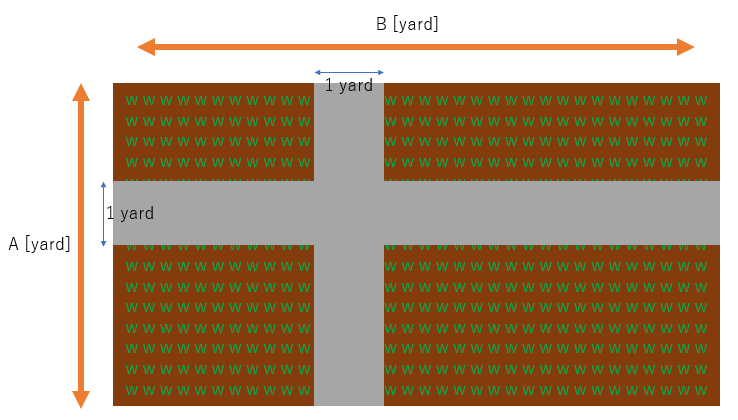﻿ ABC106 A - Garden - Atcoder

# Home

﻿Score: $100$ points

### Problem Statement

There is a farm whose length and width are $A$ yard and $B$ yard, respectively. A farmer, John, made a vertical road and a horizontal road inside the farm from one border to another, as shown below: (The gray part represents the roads.)What is the area of this yard excluding the roads? Find it.

### Note

It can be proved that the positions of the roads do not affect the area.

### Constraints

• $A$ is an integer between $2$ and $100$ (inclusive).
• $B$ is an integer between $2$ and $100$ (inclusive).

### Input

Input is given from Standard Input in the following format:

$A$ $B$


### Output

Print the area of this yard excluding the roads (in square yards).

### Sample Input 1

2 2


### Sample Output 1

1


In this case, the area is $1$ square yard.

### Sample Input 2

5 7


### Sample Output 2

24


In this case, the area is $24$ square yards.